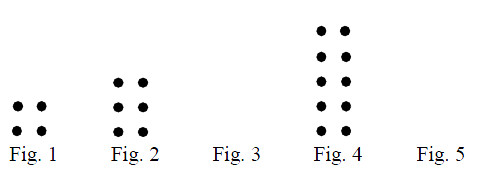### Home > MC1 > Chapter 3 > Lesson 3.2.1 > Problem3-32

3-32.Study the dot pattern below. 3-32 HW eTool (CPM). Homework Help ✎

1. Draw the 3rd and 5th figures.

Can you see a pattern in the completed figures? Use this pattern to complete figures 3 and 5.

2. How many dots will there be in the 50th figure?

The number of dots in a figure is described by the equation # of dots = 2 + 2x where x is the figure number. Plug in x = 50 to find the answer.

$102$ dots

3. Is there a figure that will have exactly 38 dots? If there is, which figure is it?

Plug in $38$ for ''# of dots'' in the equation above, then solve for x. If you get a whole number, that is the figure number.

Use the eTool below to create the patterns for Fig. 3 and Fig. 5.
Click the link at right for the full version of the eTool: MC1 3-32 HW eTool